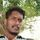cancel
Showing results for
Did you mean:Frequent Visitor

## Help with filtering/ sum by a date from a summary table

Hello,

im a new user so please exuse my basic understandings to what may be a simple problem.

My issues is this

I have 2 tables 1 is a summary table that i am summarising total per day for a month. The issue i am having is sum the total of column A, column B is the date and there re mulitple of the same day. I need to return the sum of column A based on the date that is derived from the Summary table. Any suggested will be greatly apreciated.

Thanks

Daryl

2 REPLIES 2Resolver I

Hi @Brainscand ,

Option.
go to the modeling inside new table .
Then make it inquice summarzice value.
= summarazice column ( table 1, column , column 2)..
. and  aside try to use the date formula for month. new column
= format (Date,"01-MM-YYYY"). this date formula is working by day wise data wil be calculating the month first date.

Regrds,
Gokulakannan. GSolution Sage

@Brainscand
Total hours =

SUM('ABCD - Лист1'[Hours])

Measure = SUMX(VALUES('ABCD - Лист1'[Date]),[Total hours])